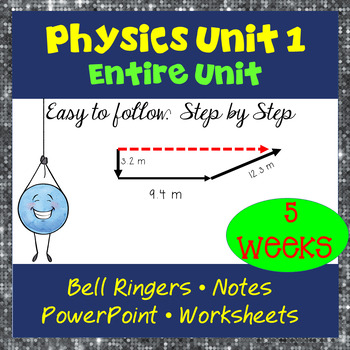# Physics Unit 1 Graphing & 1 D Kinematics: The total bundle!9th - 12th, Homeschool
Subjects
Resource Type
Formats Included
• Zip
Pages
350 pages
\$42.00
Bundle
List Price:
\$45.75
You Save:
\$3.75
\$42.00
Bundle
List Price:
\$45.75
You Save:
\$3.75

### Description

This is an incredible resource and is the first unit of my Physics Curriculum. You have your physics worksheets, bell ringers, potential quizzes, notes, student notes, PowerPoint, and a graphing match card sort. This covers everything from position-time graphs, to velocity-time, acceleration-time, distance, displacement, speed, velocity, acceleration, significant figures, and motion maps. Then it moves on to 1 Dimension kinematics and the 4 kinematics equations.

This is 5 weeks of materials.

It is TOUGH to find quality physics resources out there. Especially ones that include everything that is included in here. This will add time and sanity back to your life! :)

All answer keys included. Misconceptions explained. Everything written to unit objectives.

Check out my unit objectives:

1. I can describe mechanics and kinematics as it relates to studying physics.
2. I know what accuracy and precision mean and why both are important to science.
3. I can define vector and scalar and understand when a measurement is vector or scalar. I can also identify the key difference between vector and scalar.
4. I understand distance and displacement and understand their critical differences in physics. I can identify when to use each to solve problems.
5. I understand significant figures, the rules of how to apply them, and why they matter to scientists.
6. I can compare and contrast between speed and velocity. I can calculate each and know how they relate to distance and displacement. I know their correct units.
7. I can describe and calculate acceleration. I understand that acceleration can be positive or negative and what that means.
8. I can visually display motion using motion maps.
9. I know the proper rules for graphing
10. I can convert motion map data into a position-time graph.
11. I can calculate slope of a position-time graph and realize the slope means velocity. I know that a curved position-time graph indicates acceleration.
12. I can describe motion using a velocity-time graph. I know that slope of a velocity-time graph equals acceleration. I can convert a velocity-time graph to an acceleration-time graph
13. I can calculate area under the line of a Velocity-Time graph and know that equals displacement.
14. I can identify the variables initial velocity, final velocity, acceleration, displacement, and time. I know there are 4 main kinematics equations and I can decide when to use which equation.
15. I can use the kinematics equations to solve for a missing unknown.
16. I understand free fall and know how to solve for unknowns in free fall problems.

Feel free to email if you have any questions, concerns, or suggestions. I'm happy to help! bcsfamily@gmail.com

Total Pages
350 pages
Included
Teaching Duration
1 month
Report this Resource to TpT
Reported resources will be reviewed by our team. Report this resource to let us know if this resource violates TpT’s content guidelines.